# 1698 A Little Virgács and Candy

### Today’s Puzzle:

If you were a child in Hungary, you might have found some virgács and some candy in your boot this morning. Mikulás (St. Nick) would have given you the candy because of how good you’ve been this year, and the virgács for those times you weren’t so good.

This virgács and candy puzzle is like a mixed-up multiplication table. It is a lot easier to solve because I made it a level 3 puzzle. First, find the common factor of 56 and 72 that will allow only numbers between 1 and 12 to go in the first column. Put the factors in the appropriate cells, then work your way down the puzzle, row by row until each number from 1 to 12 is in both the first column and the top row.### Factors of 1698:

• 1698 is a composite number.
• Prime factorization: 1698 = 2 × 3 × 283.
• 1698 has no exponents greater than 1 in its prime factorization, so √1698 cannot be simplified.
• The exponents in the prime factorization are 1, 1, and 1. Adding one to each exponent and multiplying we get (1 + 1)(1 + 1)(1 + 1) = 2 × 2 × 2 = 8. Therefore 1698 has exactly 8 factors.
• The factors of 1698 are outlined with their factor pair partners in the graphic below.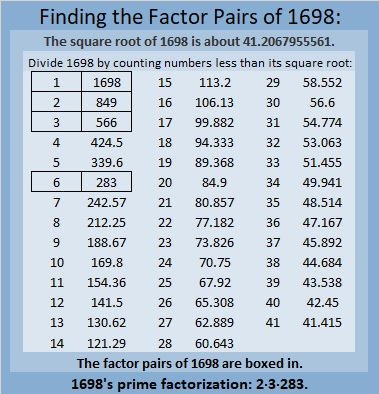### More About the Number 1698:

1698 = 2(849)(1), so it is a leg in the Pythagorean triple calculated from
2(849)(1), 849² – 1², 849² + 1².

1698 = 2(283)(3), so it is a leg in the Pythagorean triple calculated from
2(283)(3), 283² – 3², 283² + 3².

# 1561 Virgács for Boots and Stockings

### Today’s Puzzle:

Children living in Hungary put their nicely polished boots or stockings by a window for Mikulás (Saint Nicholas) to fill tonight. When they awake in the morning, they will find candies, and maybe nuts or fruit to reward them for the good they’ve done this past year. Because even the best children have been at least a little bit naughty sometime during the year, they will also find virgács, gold-painted twigs typically bound together with red ribbon. Now, if a child lives in a place where virgács is not available at the local market, Mikulás could copy today’s virgács puzzle and put it in any boot or stocking left out for him tonight.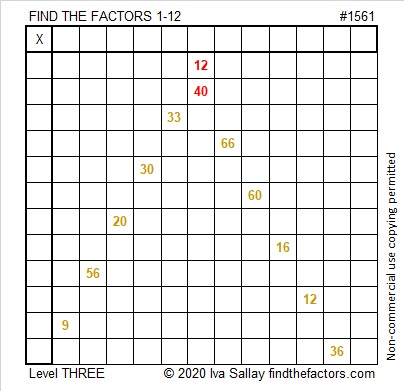Since this is a level 3 puzzle, the clues are listed in a logical order from the top of the puzzle to the bottom. After the factors of 12 and 40 are put in their respective cells, the rest of the factors can be found by working down the puzzle cell by cell until all the factors are written in.

### Factors of 1561:

• 1561 is a composite number.
• Prime factorization: 1561 = 7 × 223.
• 1561 has no exponents greater than 1 in its prime factorization, so √1561 cannot be simplified.
• The exponents in the prime factorization are 1 and 1. Adding one to each exponent and multiplying we get (1 + 1)(1 + 1) = 2 × 2 = 4. Therefore 1561 has exactly 4 factors.
• The factors of 1561 are outlined with their factor pair partners in the graphic below.### More about the Number 1561:

1561 is the sum of two consecutive numbers:
780 + 781 = 1561.

1561 is also the difference of two consecutive square numbers:
781² – 780² = 1561.

Did you notice a pattern in those two statements?

1561 is the sum of seven consecutive numbers:
220 + 221 + 222 + 223 + 224 + 225 + 226 = 1561.

1561 is the sum of the fourteen consecutive numbers from 105 to 118.

1561 is the difference of these two other square numbers:
115² – 108² = 1561.

Did you notice any other patterns? Does your pattern hold true for other multiples of 7?

# 1445 Virgács for Your Boots Tonight

Tomorrow is Mikulás (Saint Nicholas Day) in Hungary. Children will awake to find candy, fruit, or nuts in their polished shoes or boots because every boy and every girl has been at least a little bit good all year long.

Because they have also been at least a little bit naughty, they will also find virgács in those same shoes or boots. Virgács are little twigs that have been spray-painted gold and tied together at the top with red ribbon.

Santa is so busy this time of year, that I thought I would give him a helping hand. I’ve made some virgács for YOUR boots or shoes!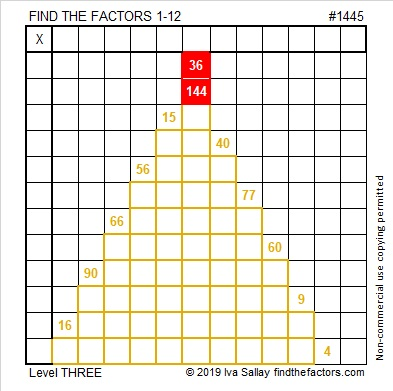Start at the top of the puzzle and work your way down cell by cell to solve this Level 3 puzzle. Oh, but I’ve been just a little bit naughty making this puzzle: you will need to look at later clues to figure out what factors to give to 40. Will clue 40 use a 5 or a 10? Look at clues 60 and 90, and you will have only one choice for that answer. Then you can forgive my tiny bit of naughtiness.

Now I’ll tell you a few facts about the puzzle number, 1445:

• 1445 is a composite number.
• Prime factorization: 1445 = 5 × 17 × 17, which can be written 1445 = 5 × 17²
• 1445 has at least one exponent greater than 1 in its prime factorization so √1445 can be simplified. Taking the factor pair from the factor pair table below with the largest square number factor, we get √1445 = (√289)(√5) = 17√5
• The exponents in the prime factorization are 1 and 2. Adding one to each exponent and multiplying we get (1 + 1)(2 + 1) = 2 × 3 = 6. Therefore 1445 has exactly 6 factors.
• The factors of 1445 are outlined with their factor pair partners in the graphic below.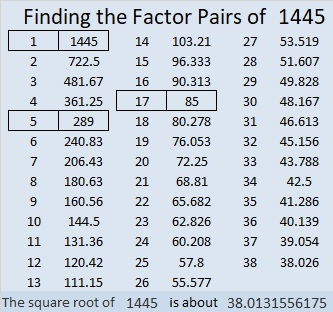1445 is the sum of two squares in THREE different ways:
31² + 22² = 1445
34² + 17² = 1445
38² + 1² = 1445

1445 is the hypotenuse of SEVEN Pythagorean triples:
76-1443-1445 calculated from 2(38)(1), 38² – 1², 38² + 1²
221-1428-1445 which is 17 times (13-84-85)
477-1364-1445 calculated from 31² – 22², 2(31)(22), 31² + 22²
612-1309-1445 which is 17 times (36-77-85)
680-1275-1445 which is (8-15-17) times 85
805-1200-1445 which is 5 times (161-240-289)
867-1156-1445 which is (3-4-5) times 289 and can
also be calculated from 34² – 17², 2(34)(17), 34² + 17²

# 701 Some Virgács left by Mikulás

• 701 is a prime number.
• Prime factorization: 701 is prime.
• The exponent of prime number 701 is 1. Adding 1 to that exponent we get (1 + 1) = 2. Therefore 701 has exactly 2 factors.
• Factors of 701: 1, 701
• Factor pairs: 701 = 1 x 701
• 701 has no square factors that allow its square root to be simplified. √701 ≈ 26.4764046.

How do we know that 701 is a prime number? If 701 were not a prime number, then it would be divisible by at least one prime number less than or equal to √701 ≈ 26.4. Since 701 cannot be divided evenly by 2, 3, 5, 7, 11, 13, 17, 19, or 23, we know that 701 is a prime number.Tonight Mikulás will visit the homes of children who sleep in Hungary. If they have been good, he will fill their boots with sweet treasures. If they have been naughty, they will receive virgács, small twigs that have been spray painted gold and bound together with red decorative ribbon. Actually most children make both the naughty list and the nice list so their boots are filled with a mixture of sweet and the not so sweet including virgács, a subtle reminder to be good.

I especially like this illustration from Wikipedia that features Mikulás (Saint Nickolas) and Krampusz:I like that it is 150 years old. It is from 1865, several decades before any of my husband’s grandparents left Hungary to live in the United States. Under the chair is a little boy hiding from Krampusz. I like to imagine he’s related to my husband some way. The little girl in the illustration must have been much better behaved that year because she is not afraid enough to need to hide.

Since everyone has been at least a little bit naughty this year, here is virgács for you to put in your shoes tonight, too.Print the puzzles or type the solution on this excel file: 12 Factors 2015-11-30

———————————————————————————

Here is a little more about the number 701:

26² + 5² = 701 so it is the hypotenuse of the primitive Pythagorean triple 260-651-701 which can be calculated using 2(26)(5), 26² – 5², 26² + 5².

701 is the sum of three consecutive prime numbers: 229 + 233+ 239.

701 is a palindrome in several bases:

• 10301 BASE 5; note that 1(625) + 0(125) + 3(25) + 0(5) + 1(1) = 701.
• 858 BASE 9; note that 8(81) + 5(9) + 8(1) = 701.
• 1F1 BASE 20; note that F is equivalent to 15 in base 10, and 1(400) + 15(20) + 1(1) = 701.
• 131 BASE 25; note that 1(625) + 3(25) + 1(1) = 701

OEIS.org informs us that 1^0 + 2^1 + 3^2 + 4^3 + 5^4 = 701.

Here’s another way we know that 701 is a prime number: Since 701 ÷ 4 has a remainder of 1, and 701 can be written as the sum of two squares that have no common prime factors (26² + 5² = 701), then 701 will be prime unless it is divisible by a primitive Pythagorean hypotenuse less than or equal to √701 ≈ 26.4. Since 701 is not divisible by 5, 13, or 17, we know that 701 is a prime number.

———————————————————————————# 317 and Candies from Mikulás

• 317 is a prime number.
• Prime factorization: 317 is prime.
• The exponent of prime number 317 is 1. Adding 1 to that exponent we get (1 + 1) = 2. Therefore 317 has exactly 2 factors.
• Factors of 317: 1, 317
• Factor pairs: 317 = 1 x 317
• 317 has no square factors that allow its square root to be simplified. √317 ≈ 17.804How do we know that 317 is a prime number? If 317 were not a prime number, then it would be divisible by at least one prime number less than or equal to √317 ≈ 17.804. Since 317 cannot be divided evenly by 2, 3, 5, 7, 11, 13, or 17, we know that 317 is a prime number.

Today is Mikulás or St. Nickolas’s name day in Hungary. Children woke up to find various candies such as the candy cane below in their shined shoes this morning.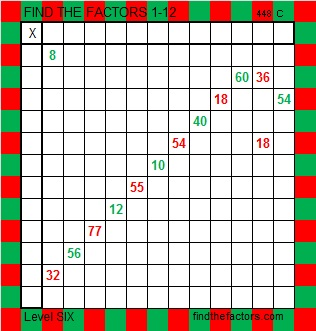Print the puzzles or type the factors on this excel file: 12 Factors 2014-12-01My grandson left this note for Mikulás last night.Köszönöm Mikulás” means “Thank you, St. Nickolas.” My grandson was thrilled with the candies and amused by the virgács that he found in his shoes this Mikulás morning.

# 312 and Virgács

Today might not be the 312th day of the year, but here’s a fun fact about the number 312:

• 312 is a composite number.
• Prime factorization: 312 = 2 x 2 x 2 x 3 x 13, which can be written 312 = (2^3) x 3 x 13
• The exponents in the prime factorization are 3, 1, and 1. Adding one to each and multiplying we get (3 + 1)(1 + 1)(1 + 1) = 4 x 2 x 2 = 16. Therefore 312 has exactly 16 factors.
• Factors of 312: 1, 2, 3, 4, 6, 8, 12, 13, 24, 26, 39, 52, 78, 104, 156, 312
• Factor pairs: 312 = 1 x 312, 2 x 156, 3 x 104, 4 x 78, 6 x 52, 8 x 39, 12 x 26, or 13 x 24
• Taking the factor pair with the largest square number factor, we get √312 = (√4)(√78) = 2√78 ≈ 17.664The night of December 5th, Mikulás, or St. Nickolas, will visit homes in Hungary, some neighboring countries, and even a few houses in the United States.  Children who have been good will awaken to find their shoes filled with little treats such as candy, fruit or nuts. Since all children are occasionally a little bit naughty, they will also find virgács, a small collection of twigs that have been spray-painted gold and decoratively bound together.  Virgács are not sold in the United States, so St Nickolas will be making some himself using the bristles from a natural broom.  The finished product should look like today’s puzzle.

This puzzle is meant to be reminiscent of the virgács my children’s ancestors probably received each year on St Nickolas Day.
Print the puzzles or type the factors on this excel file: 12 Factors 2014-12-01
A Logical Approach to FIND THE FACTORS: Find the column or row with two clues and find their common factor. Write the corresponding factors in the factor column (1st column) and factor row (top row).  Because this is a level three puzzle, you have now written a factor at the top of the factor column. Continue to work from the top of the factor column to the bottom, finding factors and filling in the factor column and the factor row one cell at a time as you go.

# 13 Mikulás

• 13 is a prime number.
• Prime factorization: 13 is prime.
• The exponent of prime number 13 is 1. Adding 1 to that exponent we get (1 + 1) = 2. Therefore 13 has exactly 2 factors.
• Factors of 13: 1, 13
• Factor pairs: 13 = 1 x 13
• 13 has no square factors that allow its square root to be simplified. √13 ≈ 3.60555.How do we know that 13 is a prime number? If 13 were not a prime number, then it would be divisible by at least one prime number less than or equal to √13 ≈ 3.6. Since 13 cannot be divided evenly by 2 or 3, we know that 13 is a prime number.

13 is never a factor in the FIND THE FACTOR 1 – 10 or 1 – 12 puzzles.

Tonight (December 5th) all over Hungary, children will polish their boots and then place them in a window or in front of their door. Once the children are “nestled, all snug in their beds, … visions of sugar-plums (will indeed) dance in their heads” as they await a visit from Mikulás, or St. Nickolas.  When the children get up in the morning, they will find their boots or shoes filled with candy, fruit, and nuts if they have been good. If they have been bad, their boots or shoes will be filled with virgács, a small collection of twigs that have been spray-painted gold and decoratively bound together.

Since most children were good some of the time and naughty once in awhile, they will likely find  the expected goodies as well as virgács in their shoes or boots.
With these traditions in mind, I created the puzzles for today. If you have just a little imagination, you will be able to see different types of candy as well as the virgács in the clues. These puzzles will be a treat to any child or adult who did their homework and learned multiplication, division, and factoring. Click 12 Factors 2013-12-05.